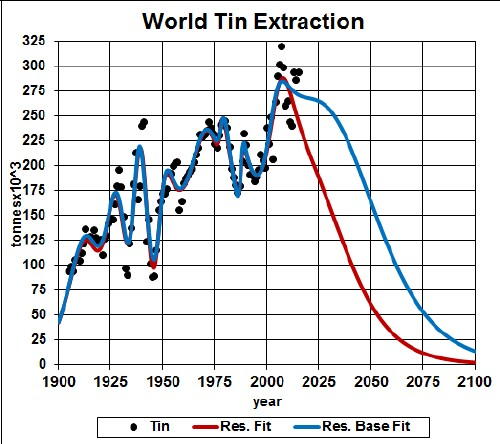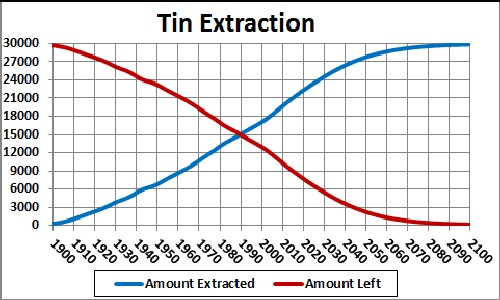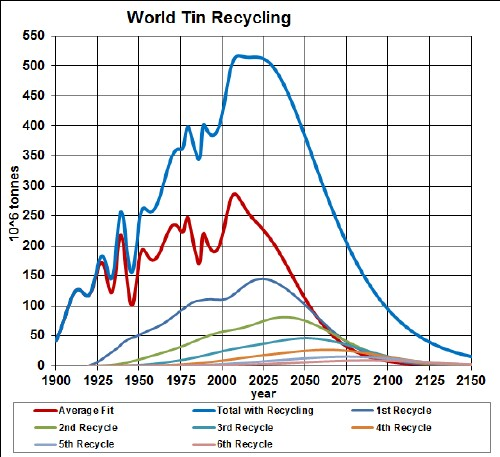# Tin Depletion

Tin is used with copper to make bronz. The graph below shows the tin extraction data for the world and two Verhulst function fits to the data in order to extrapolate into the future.Tin extraction rate for the world and Verhulst function fits to the data.

The red curve is a fit with total amount to be extracted that incorporates the latest reserves estimate (~4.8 x 106 tonnes), and the blue curve is fit with total amount to be extracted that incorporates the latest reserves-base estimate (~11 x 106 tonnes).

It appears that world-tin extraction will peak before 2025.

Taking an average extraction curve of the two fits, the crossover point at year ~1990 when the amount extracted is equal to the amount left to be extracted is shown here:## Recycling

tin, especially the tin used in batteries, is recycled to a high degree. Assume that:

• The tin-extraction curve is the average of the two curves given above.
• Recycling of tin follows a hyperbolic tangent curve from 10% to 70% recycling with a break point of year 1950 and width 50 years.
• The recycling is delayed by a Gaussian curve peaking at a delay of 10 years and a width of 10 years.

The effective tin available for making items after the first ten recycling cycles is shown in the following graph, along with the effective tin available for each cycle:The equation for a recyling cycle is,

where Ei is the amount available from the previous cycle. Here is an example of the Excel coding:

{=((\$J\$2+\$I\$2)/2+((\$J\$2-\$I\$2)/2)*TANH((A27-\$K\$2)/\$L\$2))*SUM(\$I\$27:I27*(EXP(-1*((A27-\$A\$27:A27-\$N\$2)/\$O\$2)^2/2))/\$O\$2/SQRT(2*PI()))} (The curly bracket surrounding the term makes it into an array; it must be entered by holding down the SHIFT & CTRL keys while pressing the ENTER key.)

Of course, the recycling could be extended to more cycles, skewing the curve further into the future. However, the peak and fall off after it will not change because further cycles are essentally zero in that time region.

Thus, under the assumptions given above, the effective amount of tin available for making items peaks at about 2020 and falls off rapidly after that. Humans will have taken concentrated tin deposits and scattered them across the surface of the earth.

The Excel spreadsheet is set up to make it easy to calculate with different recycling assumptions.

Minerals Depletion